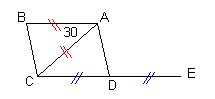Question from Sam, in middle school: Parallelogram ABCD has diagonal AC equal in length to side AB. CD is produced to E so that D is between E and C. If angle BAC =30 degrees find the size of angle ADE. Hi Sam.I drew a diagram fitting your description on the right. You have to think first about the properties of parallelograms. What is the relationship between the lengths of the sides AB and CD? Then ask what is the relationship between the angle BAC and the angle ACE. Remember that a line that crosses a pair of parallel lines creates matching angles. The third thing you need to think about is what kind of triangle is ACD. If you know the angle ACE, then the type of triangle should tell you the measure of the angle ADC. The last step is to remember that supplementary angles ADC and ADE add to 180 degrees. Hope this helps! Stephen La Rocque.